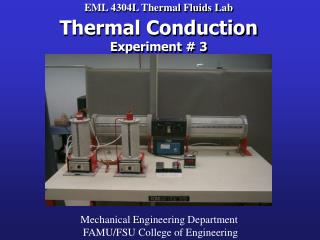DownloadDownload PresentationEML 4304L Thermal Fluids Lab Thermal Conduction Experiment # 3

# EML 4304L Thermal Fluids Lab Thermal Conduction Experiment # 3

Download Presentation## EML 4304L Thermal Fluids Lab Thermal Conduction Experiment # 3

- - - - - - - - - - - - - - - - - - - - - - - - - - - E N D - - - - - - - - - - - - - - - - - - - - - - - - - - -
##### Presentation Transcript

1. EML 4304L Thermal Fluids LabThermal ConductionExperiment # 3 Mechanical Engineering Department FAMU/FSU College of Engineering

2. Outline • Purpose of the lab • Fundamental Equations • Unit 3 and Unit 4 Analysis • Unit 1 and Unit 2 Analysis • Error Analysis

3. PURPOSE • Conduct a series of thermal conduction experiments which examines the effects on heat transfer with varying cross-sectional area and distance. • Using this thermal conduction information derive Fourier’s law of thermal conduction. • Analyze the temperature variance in a series of metal rods that are in physical contact. • From this information determine thermal resistance and contact resistance.

4. Q coolant=m Cp dT / t Q conduction=-k A dT / dx Heat flow for units 3 and 4

5. Heat flow for units 1 and 2

6. Fundamental Equations

7. Rate of heat flow at the heat sink mw = mass of cooling water displaced in time t (kg) Cp = Specific heat of water at constant pressure (kJ/kg °C) T = (Tout - Tin) of cooling water (°C) t = time required to displace a volume Vw of water (s) This equation is used to determine the amount of energy that is being absorbed by the coolant. Once this is determined for each unit, it is assumed to be the constant rate of conduction through each material.

8. Qcond = KA(ΔT/Δx) Rate of heat conduction K= thermal conductivity constant (W/m °C) A = cross-sectional area (m2) T = temperature difference across the material (°C) x = distance between temperature readings (m) Used to determine rate of heat conduction through a body based on material properties, area, temperature difference, and length of material.

9. Qcond = -KA dT/dx Fourier’s law of heat conduction -K = thermal conductivity constant (W/m °C) A = cross-sectional area (m2) dT = differential element for temperature (°C) dx = differential element for distance (m) Used to determine rate of heat conduction through a body based on material properties, area, and temperature/distance gradient.

10. Q=KA ΔT/Δx Q=-KA dT/dx • dT/dx = temperature gradient T dT/dx x

11. Calculations Conservation of Energy Qin = Q out Q conduction = Q coolant This equation assumes there is no heat loss through the system boundary. Though each unit is insulated, there will still be some heat loss.

12. Thermal Contact Resistance Rt,c = Thermal contact resistance (ºC/W) T = Temperature change (ºC) q = Heat flux (W) Calculates thermal contact resistance for a given temperature discontinuity and a known power input.

13. Heat Conduction for Units 3 and 4

14. Thermocouple Placement for Units 3 and 4

15. UNIT #3 Q=KA*ΔT/Δx Diameter is a function of x: D(x)=D0+mx D(x)=1”+(x/(11+1/16))

16. Unit #3 Area can also be written as a function of x: A = (p/4) d2 A(x) = (p/4) d(x)2 Q=KA*dT/dx

17. Unit #3 Q = -k A DT/Dx = -k A(x) dT/dx

18. Unit #3 Once k is solved for, the temperature can be found for any distance, x.

19. UNIT #4 Q=KA*ΔT/Δx K = coeff. of therm. conductivity NOTE: K is unknown and must be determined Qin Qout x2 x1

20. Thermal Contact Resistance Determination Units #1 and #2

21. Thermal contact resistance(Rc) is a discontinuity in the temperature gradients between two materials in contact. The value is determined by projecting the temperature gradients, calculating the temperature difference, and dividing the temperature difference by the power that is transmitted through the materials. Factors affecting thermal contact resistance: 1 - Surface Roughness 2 - Type of materials in contact 3 - Temperature materials are at 4 - Pressure applied to materials 5 - Type of fluid trapped at interface

22. elements are enclosed in the insulating jacket. Figure1 illustrates the schematics of the apparatus. The dimensions of the tapered rod are indicated in Fig. 2. elements are enclosed in the insulating jacket. Figure1 illustrates the schematics of the apparatus. The dimensions of the tapered rod are indicated in Fig. 2. elements are enclosed in the insulating jacket. Figure1 illustrates the schematics of the apparatus. The dimensions of the tapered rod are indicated in Fig. 2.

23. Units #1 and #2 Steel (Mg) Cu (Al) Stainless Steel T10 T1 x2 x1 Contact Resistance

24. Thermocouple placement for units 1 and 2

25. Ideal Thermal Conduction Material 1 Material 2 T1 T3 T4 T2 T2 = T3

26. Actual Thermal Conduction Material 1 Material 2 T2 T1 T4 Temperature profile due to thermal contact resistance T3 T2 = T3

27. Material 1 Material 2 Projected Slope T2 T2 ΔT Temperature profile due to thermal contact resistance Projected Slope T3 T3

28. Temperature vs Distance Material 1 Material 2 Temperature(ºF) Material 3 Discontinuities where ΔT must be determined Distance(inches)

29. Thermal Contact Resistance Calculation Rt,c = ΔT/Q Q = Qwtr Qwtr=mwCp(ΔT) ΔT (determined by projection of slope and measuring difference in temperatures)

30. Errors • Time Flow rate Steady State • Heat Losses Not perfectly insulated • Unit #4 Thermocouples #3 and #5 Inconsistent readings

31. Heat flow for units 3 and 4